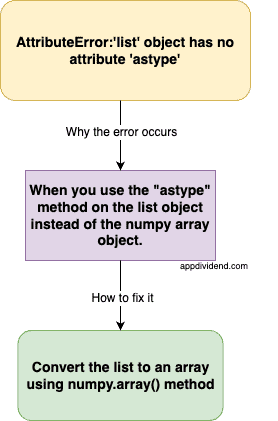# How to Fix AttributeError:’list’ object has no attribute ‘astype’

AttributeError:’list’ object has no attribute ‘astype’ error occurs because you try to operate the “astype” method on the list object instead of the numpy array object.

The astype() method is associated with NumPy arrays and is used to change the data type of the elements in the array.

## Overview of the error and solution## Reproduce the error

``````# Convert list to numpy array
my_list = [1, 2, 3, 4]

print(my_list.astype(float))
``````

Output

``````AttributeError: 'list' object has no attribute 'astype'
``````

## How to fix the error?

To fix the AttributeError:’list’ object has no attribute ‘astype’ error, convert the list to an array using numpy.array() method and then use the astype() method on the numpy array object.

``````import numpy as np

# Convert list to numpy array
my_list = [1, 2, 3, 4]

# Converting a list to numpy array
numpy_array = np.array(my_list)

print(numpy_array.astype(float))``````

Output

``````[1. 2. 3. 4.]
``````

In this example, we converted the integers in the list to floats using the astype() method on a NumPy array.

If you want to use a different data type, simply replace float with your desired type, such as int, str, etc.

You can see that we successfully fixed the error.

This site uses Akismet to reduce spam. Learn how your comment data is processed.